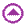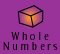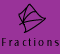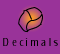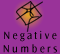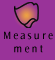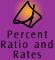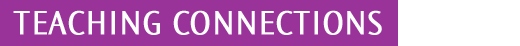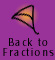| Teaching connections for fractions | Using Models |
| Identifiying the unit | Operations |

Teaching Connections for fractions

The fractions section on the CD-ROM is fairly comprehensive and provides a general sequential approach which could be used to teach fractions in the primary and secondary classroom. Where we have used pictorial representations of models to illustrate concepts, concrete models would be used in the classroom.

In the early years of primary school, fractions are commonly used when talking about food, such as 'I've eaten half of my sandwich' and in numbers as in, 'Half the class is going to music now'. Children readily identify with a half and teachers should be aware that anything a child can do with a half says little about what they can do with other fractions. A quarter is the next fraction children learn, and they tend to think of it initially as a half of a half rather than something divided into 4. By around Year 2, children should be able to divide a rectangle into 3 equal parts confidently. Up to this point, their tendency is to divide an object successively into 2 equal parts.

Although fractions are used loosely in natural language, teachers need to stress right from the beginning that when we are doing something mathematically with fractions the fractional parts have to be exactly equal. Also at around Years 3 and 4 children begin ordering and comparing fractions, initially by using equivalent fractions. A fraction wall (see below) is a useful illustration of the fact that when the denominator of a fraction goes up the size goes down.

The sizes of fractions can be modelled by lengths or areas provided it is clear what the unit is.

Using Models

- Use fraction kits. Use computer software to show fractional parts and equivalence.

- Establishing the notion of equivalence requires lots of work. It is important that you take the time to develop the notion that there are many names for the one fraction.

- Work in simple fractions that are familiar to the students when first carrying out operations. This will allow the students more of a chance to develop a deeper understanding than they do with more complicated fractions where they have a tendency to work the calculations by recipes and lose understanding of the process.

- Use real life contexts for problems.

- Fractions can be modelled using length (e.g. fraction wall), area of regions (e.g. fraction kits) or numbers of objects in sets.

- Use contiguous and non-contiguous models to emphasise the fact that it is the sum of the parts / area of shading that matter(s) not the arrangement of the parts.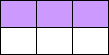In each of the rectangular objects above, the shaded area represents half the area of the object

- When using sets of objects, use both homogeneous and non-homogeneous items so that children understand that all the objects don't have to be the same. For example, a set of animals may contain all cats or may be made up of cats, dogs and elephants etc. If we want to find one third of the number of animals, it doesn't matter what type of animals they are.

Appropriate concrete models include:

 fraction kits fraction strips fraction towers fraction walls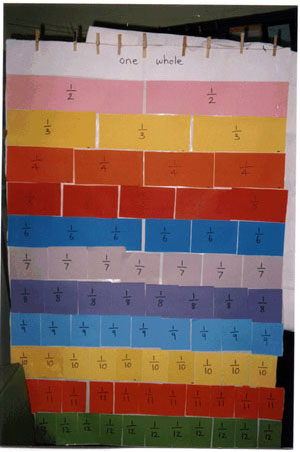A fraction wall made in the classroom (Kate Hughes kindly gave us permission to use this picture)

 While concrete materials have a very important role to play, teachers need to be aware that continued adherence to concrete models can cause children, and eventually adults, to be unable to recognise fractions as numbers in their own right. For example, -1/2 cannot exist when working with concrete materials and some children continue to believe that it can therefore not exist as a number.

Fractions are first taught as a description of quantities or an operator on quantities. For example, one fifth of the distance from here to Mooroolbark describes a distance in relation to another distance. However, fractions also have to come to be seen as numbers. It is very important that children gradually build up the idea of one number line which holds all real numbers, otherwise children may have the misconception that decimals fit on one number line, whole numbers on another and fractions on another. Children need to see that all these numbers can be accommodated on the one number line.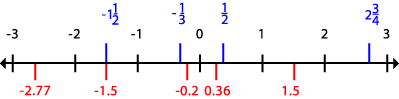Identifying the unit

Children (and the teacher) must always have a clear understanding of what the unit is, ie. what represents 1. Keep referring to the unit to ensure children do not lose sight of it. Be aware of drawing misleading illustrations which could confuse children.

For example,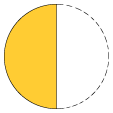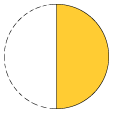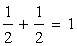In this example the teacher has drawn a dashed line to indicate where the missing half of the circle lies. However it also looks like 4 half circles have been drawn. This example could be misconstrued to show that 1/2 + 1/2 = 2/4.

Operations

By the end of primary school we expect children to be able to

- add and subtract fractions with like denominators

- add and subtract fractions with related denominators (see movies)

- add fractions with unrelated denominators by finding a common denominator but not necessarily by using the lowest common denominator.

For example, children might find a common denominator by generating equivalent fractions or by multiplying the denominators together.

Fraction addition and subtraction algorithms can be usefully taught by writing a mix of symbols and words in the early stages. For example, it can be less confusing for beginners to think about

7 eighths - 2 eighths = 5 eighths than

7/8 - 2/8 = 5/8.

Multiplication and Division

With fraction algorithms, the concrete materials don't necessarily reinforce the algorithm steps. We can illustrate the meaning of 1/2 of a 1/4 with materials but we can't show why we multiply 1/2 by 1/4 until later primary years where an area model can be used. Children need to understand the properties of numbers in order to see why the answer is what it is.

For example: 1/2 of 1/4 can be approached in a couple of ways depending on the stage of development. In the earlier primary years a fraction pieces model could be used, as below.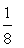This model shows that 1/2 of 1/4 equals 1/8 but it does not show that 1/2 x 1/4 = 1/8. Here the 1/2 is acting as an operator on the 1/4, i.e. we have a quarter and if we take a half of the quarter then we have an eighth.

In order to link of to x children need to build on whole numbers' work to develop their understanding of properties of numbers.

For example,

2 lots of 4 donuts = 8 donuts, or we could say,

2 servings of 4 donuts

3 servings of 4 donuts = 12 donuts,

1/2 serving of 4 donuts = 2 donuts,

1/2 serving of 1 donut = 1/2 of a donut,

1/4 of a donut = 1/8 of a donut

In late primary school children will be familiar with the formula for the area of finding a rectangle. By generalising from whole number work, the area model can be applied to fractions.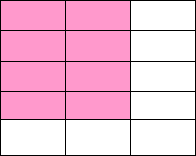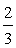4/5 x 2/3 = (4 x 2)/(5x3) = 8/15

This model demonstrates the algorithm; it shows that we can obtain the answer by multiplying the numerators together and the denominators together.

The rationale for teaching the fraction algorithms is not just to do calculations, but also because fraction notation is used as the notation for division in algebra, eg. x/y.

Some curriculum documents do not require that children learn the algorithms for multiplication and division. However, by the end of primary school children should be able to do simple questions such as these:

What is a 1/3 of 63? (i.e. link multiplication by a fraction to division by the reciprocal)

How many 1/2 are in 1 1/2? (i.e. mental quotition division in simple cases)

Share 1 1/2 in 3 equal pieces? (i.e. mental partition division in simple cases)

Children need to be familiar with these types of examples, which can be solved by thinking about the meaning of the question) before going onto further work with algorithms.